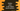# Python program to print a star hollow square pattern## Python program to print a hollow square pattern of star:

In this tutorial program, we will learn how to print a hollow square pattern in python. We will show you how to print that pattern using star (*), but you can also modify the program to print the pattern using any other character or symbol like &,%,\$,#,@, etc.

To print the pattern, we can either use for loop or while loop. We will show you how to write the code using both ways. The final output will look as like below :

``````* * * * * *
*         *
*         *
*         *
*         *
* * * * * *``````

We will ask the user to enter the size of one side of the square. Since all sides are equal, we will write the program to read one side and print the hollow square using the user-provided size.

``````length = int(input("Enter the side of the square  : "))

for i in range(length):
for j in range(length):
if(i == 0 or i == length - 1 or j == 0 or j == length - 1):
print('*', end = ' ')
else:
print(' ', end = ' ')
print()``````

### Sample output :

``````Enter the side of the square  : 6
* * * * * *
*         *
*         *
*         *
*         *
* * * * * *``````

### Explanation :

To learn how the program worked, let me change the print statement of the else statement as below :

``````length = int(input("Enter the side of the square  : "))

for i in range(length):
for j in range(length):
if(i == 0 or i == length - 1 or j == 0 or j == length - 1):
print('*', end = ' ')
else:
print('\$', end = ' ')
print()``````

Now, if you run the program, it will print a square of size like below :

``````Enter the side of the square  : 6
* * * * * *
* \$ \$ \$ \$ *
* \$ \$ \$ \$ *
* \$ \$ \$ \$ *
* \$ \$ \$ \$ *
* * * * * *``````

As you can see, the second print statement is actually used to print the blank spaces , in this example, we are using that print statement to print the \$ symbol.

• The outer print is used to print the ’*’ and the inner print is used to print the blank spaces.
• The outer loop will run for length number of times. In this example, it runs for i = 0 to i = 6. The inner loop will also run for the same time , i.e. j = 0 to j = 6.
• We are printing the * only if i == 0 , i == length - 1 , j == 0 or j == length - 1. i.e. we are printing symbol for the first row, last row, first column and last column. For other values, we are printing blank spaces.

I hope that you have got an understanding of how the program actually works and how it printed out the result. Now, let’s try to implement this using a while loop :

Same as the above example, we can also implement it using a while loop. Let me show you how :

``````length = int(input("Enter the side of the square  : "))

row = 1

while(row <= length):
column = 1;
while(column <= length ):
if(row == 1 or row == length or column == 1 or column == length):
print('*', end = ' ')
else:
print(' ', end = ' ')
column = column + 1
row = row + 1
print()``````

### Sample Output :

``````Enter the side of the square  : 6
* * * * * *
*         *
*         *
*         *
*         *
* * * * * *``````

This is same as the above example we have used with the for loop. We are just using while instead of for.

## Taking custom user input :

Now, let’s modify the above program to take user input for the character. The user will enter the character that we will use to print the square :

``````length = int(input("Enter the side of the square  : "))
ch = input("Enter the character to use in the square : ")

for row in range(length):
for column in range(length):
if(row == 0 or row == length - 1 or column == 0 or column == length - 1):
print(ch, end = ' ')
else:
print(' ', end = ' ')
print()``````

### Output :

``````@ @ @ @ @ @ @
@           @
@           @
@           @
@           @
@           @
@ @ @ @ @ @ @``````